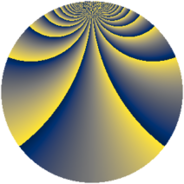# Properties

 Label 1210.2.qLevel $1210$ Weight $2$ Character orbit 1210.q Rep. character $\chi_{1210}(43,\cdot)$ Character field $\Q(\zeta_{44})$ Dimension $1320$ Sturm bound $396$

# Related objects

## Defining parameters

 Level: $$N$$ $$=$$ $$1210 = 2 \cdot 5 \cdot 11^{2}$$ Weight: $$k$$ $$=$$ $$2$$ Character orbit: $$[\chi]$$ $$=$$ 1210.q (of order $$44$$ and degree $$20$$) Character conductor: $$\operatorname{cond}(\chi)$$ $$=$$ $$605$$ Character field: $$\Q(\zeta_{44})$$ Sturm bound: $$396$$

## Dimensions

The following table gives the dimensions of various subspaces of $$M_{2}(1210, [\chi])$$.

Total New Old
Modular forms 4040 1320 2720
Cusp forms 3880 1320 2560
Eisenstein series 160 0 160

## Trace form

 $$1320q - 8q^{5} + O(q^{10})$$ $$1320q - 8q^{5} + 44q^{10} + 12q^{11} + 88q^{13} + 8q^{15} + 132q^{16} + 84q^{22} + 8q^{23} + 8q^{25} + 8q^{26} + 16q^{31} - 4q^{33} + 116q^{36} - 124q^{37} - 108q^{38} - 40q^{42} - 32q^{45} - 16q^{47} + 352q^{51} - 64q^{53} - 72q^{55} - 8q^{56} - 32q^{58} - 16q^{60} + 220q^{63} - 16q^{66} + 192q^{67} + 88q^{70} - 16q^{71} + 96q^{75} - 44q^{76} - 16q^{77} + 16q^{78} + 8q^{80} - 1320q^{81} + 16q^{82} + 176q^{85} + 64q^{86} - 4q^{88} - 232q^{91} + 36q^{92} + 56q^{93} + O(q^{100})$$

## Decomposition of $$S_{2}^{\mathrm{new}}(1210, [\chi])$$ into newform subspaces

The newforms in this space have not yet been added to the LMFDB.

## Decomposition of $$S_{2}^{\mathrm{old}}(1210, [\chi])$$ into lower level spaces

$$S_{2}^{\mathrm{old}}(1210, [\chi]) \cong$$ $$S_{2}^{\mathrm{new}}(605, [\chi])$$$$^{\oplus 2}$$